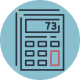0
1 Comment

Hi, I am trying to set up a calculator for this simple equation:

let result =x*(1-x);
y = roundval(result, 2);

x is a dollar amount, x is a percentage, and y is a dollar amount.

The result is set as a field (I tried both other type of results, too).  The result doesn’t show up on the webpage:

https://cashblog.com/?p=35893&preview=true

Only the first two input fields show up (x and x).  Any idea how to solve this?

CalcHub Posted new comment February 24, 2023Hello.